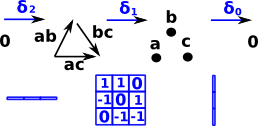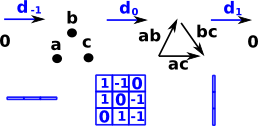# Cohomology Reversing arrows

One way to introduce cohomology is to start with homology and reverse the arrows. Can we begin with a chain complex consisting of a sequence of matrices and invert these matrices to get a cochain complex?

There are lots of problems with this:

• The matrices are not all square so to find inverse we have to choose left or right inverse if they exist.
• Matrix inverse involves division by determinant, division may not exist in integers so we would have to go to matrix over rationals.
• Determinant of boundary function tends to be zero, see case below.
 As an example lets use the chain complex of a flat disk: The determinant of this matrix is zero so we can't invert it.So the inverse arrows are not just free groups which can be represented by matrices as with homology.

### Reversing the Arrows

In the above diagram the boundary map goes from lines to points for example. each line maps to exactly 2 points.

 When we reverse the direction of the boundary map then each point maps to multiple lines.So we transpose the matrix rather than invert it.